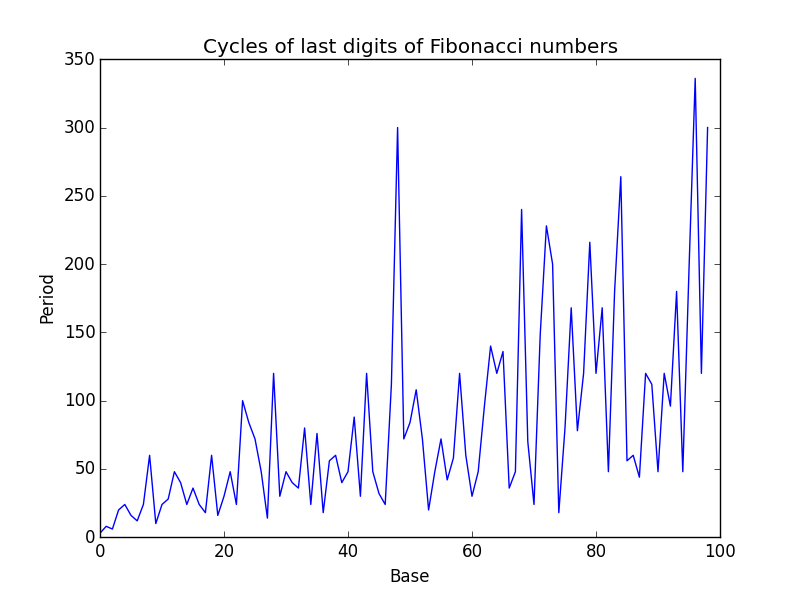# Distribution of Fibonacci numbers mod m

The last digits of Fibonacci numbers repeat with period 60. This is something I’ve written about before.

The 61st Fibonacci number is 2504730781961. The 62nd is 4052739537881. Since these end in 1 and 1, the 63rd Fibonacci number must end in 2, etc. and so the pattern starts over.

It’s not obvious that the cycle should have length 60, but it is fairly easy to see that there must be a cycle.

In any base, the last digits must cycle. The length of these cycles varies erratically:In this post I want to as a different question: how often do Fibonacci numbers take on each of the possible last digits in each base? In other words, how are the Fibonacci numbers distributed mod m for various values of m?

I haven’t tried to prove any theorems. I’ve just run some examples using the following Python code.

```    def histogram(base):

prev = 1
F = 2

counts = *base
counts[F] += 1

while F != 1 or prev != 1:
temp = F
F = (F + prev) % base
counts[F] += 1
prev = temp

return counts
```

In base 10, the last digits of Fibonacci numbers have period 60. Do all digits appear in the cycle? Do they all appear equally often?

Yes and no. Here are the results for base 10:

```    >>> histogram(10)
>>> [4, 8, 4, 8, 4, 8, 4, 8, 4, 8]
```

Each even digits appears 4 times and each odd digit appears 8 times.

What happens if we look at the last two digits, i.e. if we look at Fibonacci numbers mod 100?

```    >>> histogram(100)
>>> [2, 6, 2, 2, …, 2, 6, 2, 2]
```

Each two-digit number n appears six times if n = 1 mod 4. Otherwise it appears two times.

What about the last three digits? Now we see some zeros. For example, no Fibonacci number is congruent to 4 or 6 mod 1000. (Or mod 8.)

```    >>> histogram(1000)
>>> [2, 3, 2, 1, 0, 3, 0, 1, …, 2, 3, 2, 1, 0, 3, 0, 1]
```

Here’s something curious. The Fibonacci numbers are exactly evenly distributed mod 5.

```    >>> histogram(5)
>>> [4, 4, 4, 4, 4]
```

The same is apparently true for all powers of 5. Not only are all the frequencies the same, they’re all 4’s. I’ve tested this for powers of 5 up to 510. And conversely, it seems the Fibonacci numbers are not evenly distributed mod m unless m is a power of 5. I’ve tested this for m up to 100,000.

Conjecture: The Fibonacci numbers are uniformly distributed mod m if and only if m is a power of 5.

Update: The conjecture is correct, and was proved in 1972 by Niederreiter.

## One thought on “Distribution of Fibonacci numbers mod m”

1. Andy Jones

I’m sure there are a bunch of replies in moderation saying this, but I expect this is a consequence of the closed-form expression for the Fibonacci sequence, which has a bunch of sqrt-5s in it:

https://en.wikipedia.org/wiki/Fibonacci_number#Closed-form_expression

If you expand Binet’s formula using the binomial series, you see that the nth Fibonacci number has a residue of 1/2^(n-1) modulo 5. My number theory is rusty, but my intuition is that because multiplication over the integers modulo 5 forms a group, this residue should cycle over the group’s elements.